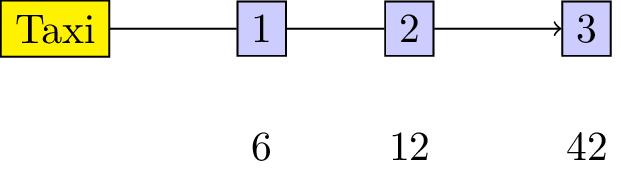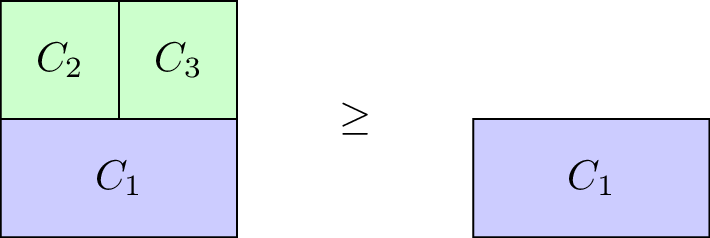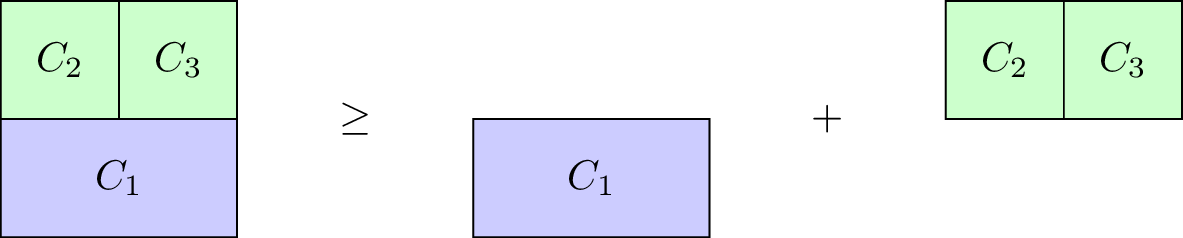## Recap

In the previous chapter:

• We defined matching games;
• We described the Gale-Shapley algorithm;
• We proved certain results regarding the Gale-Shapley algorithm.

In this Chapter we’ll take a look at another type of game.

## Cooperative Games

In cooperative game theory the interest lies with understanding how coalitions form in competitive situations.

### Definition of a characteristic function game

A characteristic function game G is given by a pair $$(N,v)$$ where $$N$$ is the number of players and $$v:2^{[N]}\to\mathbb{R}$$ is a characteristic function which maps every coalition of players to a payoff.

Let us consider the following game:

“3 players share a taxi. Here are the costs for each individual journey:

• Player 1: 6
• Player 2: 12
• Player 3: 42 How much should each individual contribute?”

This is illustrated.To construct the characteristic function we first obtain the power set (ie all possible coalitions) $$2^{{1,2,3}}=\{\emptyset,\{1\},\{2\},\{3\},\{1,2\},\{1,3\},\{2,3\},\Omega\}$$ where $$\Omega$$ denotes the set of all players ($$\{1,2,3\}$$).

The characteristic function is given below:

### Definition of a monotone characteristic function game

A characteristic function game $$G=(N,v)$$ is called monotone if it satisfies $$v(C_2)\geq v(C_1)$$ for all $$C_1\subseteq C_2$$.Our taxi example is monotone, however the $$G=(3,v_1)$$ with $$v_1$$ defined as:

is not.

### Definition of a superadditive game

A characteristic function game $$G=(N,v)$$ is called superadditive if it satisfies $$v(C_1\cup C_2)\geq v(C_1)+v(C_2).$$ for all $$C_1\cap C_2=\emptyset$$.Our taxi example is not superadditive, however the $$G=(3,v_2)$$ with $$v_2$$ defined as:

is.

## Shapley Value

When talking about a solution to a characteristic function game we imply a payoff vector $$\lambda\in\mathbb{R}_{\geq 0}^{N}$$ that divides the value of the grand coalition between the various players. Thus $$\lambda$$ must satisfy:

Thus one potential solution to our taxi example would be $$\lambda=(14,14,14)$$. Obviously this is not ideal for player 1 and/or 2: they actually pay more than they would have paid without sharing the taxi!

Another potential solution would be $$\lambda=(6,6,30)$$, however at this point sharing the taxi is of no benefit to player 1. Similarly $$(0,12,30)$$ would have no incentive for player 2.

To find a “fair” distribution of the grand coalition we must define what is meant by “fair”. We require four desirable properties:

• Efficiency;
• Null player;
• Symmetry;

### Definition of efficiency

For $$G=(N,v)$$ a payoff vector $$\lambda$$ is efficient if:

### Definition of null players

For $$G(N,v)$$ a payoff vector possesses the null player property if $$v(C\cup i)=v(C)$$ for all $$C\in 2^{\Omega}$$ then:

### Definition of symmetry

For $$G(N,v)$$ a payoff vector possesses the symmetry property if $$v(C\cup i)=v(C\cup j)$$ for all $$C\in 2^{\Omega}\setminus\{i,j\}$$ then:

For $$G_1=(N,v_1)$$ and $$G_2=(N,v_2)$$ and $$G^+=(N,v^+)$$ where $$v^+(C)=v_1(C)+v_2(C)$$ for any $$C\in 2^{\Omega}$$. A payoff vector possesses the additivity property if:

We will not prove in this course but in fact there is a single payoff vector that satisfies these four properties. To define it we need two last definitions.

### Definition of predecessors

If we consider any permutation $$\pi$$ of $$[N]$$ then we denote by $$S_\pi(i)$$ the set of predecessors of $$i$$ in $$\pi$$:

For example for $$\pi=(1,3,4,2)$$ we have $$S_\pi(4)=\{1,3\}$$.

### Definition of marginal contribution

If we consider any permutation $$\pi$$ of $$[N]$$ then the marginal contribution of player $$i$$ with respect to $$\pi$$ is given by:

We can now define the Shapley value of any game $$G=(N,v)$$.

### Definition of the Shapley value

Given $$G=(N,v)$$ the Shapley value of player $$i$$ is denoted by $$\phi_i(G)$$ and given by:

As an example here is the Shapley value calculation for our taxi sharing game:

For $$\pi=(1,2,3)$$:

For $$\pi=(1,3,2)$$:

For $$\pi=(2,1,3)$$:

For $$\pi=(2,3,1)$$:

For $$\pi=(3,1,2)$$:

For $$\pi=(3,2,1)$$:

Using this we obtain:

Thus the fair way of sharing the taxi fare is for player 1 to pay 2, player 2 to pay 5 and player 3 to pay 35.